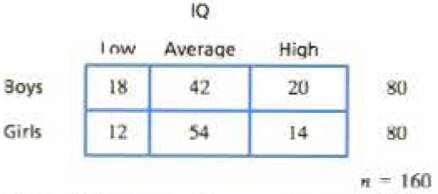Chapter 15, Problem 17PEssentials of Statistics for the B...

8th Edition
Frederick J Gravetter + 1 other
ISBN: 9781133956570

Solutions

Chapter
SectionEssentials of Statistics for the B...

8th Edition
Frederick J Gravetter + 1 other
ISBN: 9781133956570
Textbook Problem

Research results suggest that IQ scores for boys are more variable than IQ scores for s girls (Arden & Plomin, 2006). A typical study looking at 10-year-old children classifies participants by gender and by low, average, or high IQ. Following are hypothetical data representing the research results. Do the data indicate a significant difference between the frequency distributions for males and females? Test at the 05 level of significance and describe the difference.To determine

To check: Whether the data indicate a significant difference between the frequency distribution for males and females for the given question.

Explanation

Given info:

A sample of 160 students was involved in a study based on “the relation between the gender and the IQ level”. Use α=0.05 to test the claim.

Calculations:

Step 1: Null Hypothesis and Alternate Hypothesis are:

H0: No significant difference between the frequency distribution for males and females

H1: Significant difference between the frequency distribution for males and females

Step 2: For the given sample, degrees of freedom equals:

df=(R1)(C1)  where R equals number of rows and C equals columns=(21)(31)=2

With α=0.05 and df=2 , the critical value (CV)  is obtained from the χ2table as

χ2=5.991

Step 3: χ2statistics is calculated as:

χ2=(fofe)2fe

The formula to calculate expected frequency is:

fe=fcfrnwhere fr is row frequency and fc is column frequency

Substitute n=160 in the above formula and compute respective values of expected frequencies:

For the category boys, the expected frequencies are:

fe,low=30×80160fe,medium=96×80160fe,high=

Still sussing out bartleby?

Check out a sample textbook solution.

See a sample solution

The Solution to Your Study Problems

Bartleby provides explanations to thousands of textbook problems written by our experts, many with advanced degrees!

Get Started

Symmetry Test the equation for symmetry. 106. x = y4 y2

Precalculus: Mathematics for Calculus (Standalone Book)

Write the number in the form a + bi. 45. e2+i

Single Variable Calculus: Early Transcendentals, Volume I

In Exercises 49-62, find the indicated limit, if it exists. 54. limb3b+1b+3

Applied Calculus for the Managerial, Life, and Social Sciences: A Brief Approach

The graph of f(x) = 3x5 − 5x3 is:

Study Guide for Stewart's Single Variable Calculus: Early Transcendentals, 8th